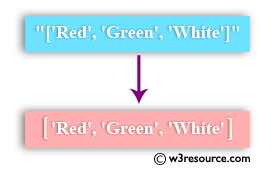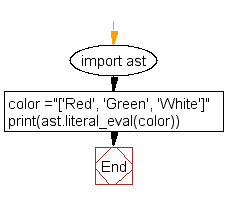﻿ Python: Convert a string to a list - w3resource# Python: Convert a string to a list

## Python List: Exercise - 56 with Solution

Write a Python program to convert a string to a list.Sample Solution:-

Python Code:

``````import ast
color ="['Red', 'Green', 'White']"
print(ast.literal_eval(color))
```
```

Sample Output:

```['Red', 'Green', 'White']
```

Flowchart:Python Code Editor:

Have another way to solve this solution? Contribute your code (and comments) through Disqus.

What is the difficulty level of this exercise?

Test your Python skills with w3resource's quiz

﻿

## Python: Tips of the Day

Floor Division:

When we speak of division we normally mean (/) float division operator, this will give a precise result in float format with decimals.

For a rounded integer result there is (//) floor division operator in Python. Floor division will only give integer results that are round numbers.

```print(1000 // 300)
print(1000 / 300)```

Output:

```3
3.3333333333333335```